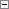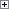This topic provides a complete overview of type conversion rules for the arithmetic operators.

Unary Arithmetic Operators

The unary arithmetic operators in C/AL are prefix operators with the following syntax.Copy Code
PrefixExpression = PrefixOperator Expression

The following table shows the data types for which the unary operators in C/AL are defined, and the resulting data types.

Unary operator Option Integer Decimal

+

integer

integer

decimal

-

integer

integer

decimal

Binary Arithmetic Operators

The following table shows the data types for which the binary arithmetic operators are defined. The binary arithmetic operators in C/AL are all infix operators.Copy Code
InfixExpression = LeftExpression InfixOperator RightExpression
Operator Boolean Byte Char Option Integer Decimal Date Time Text Code

+

No

Yes

Yes

Yes

Yes

Yes

Yes

Yes

Yes

Yes

-

No

Yes

Yes

Yes

Yes

Yes

Yes

Yes

No

No

*

No

Yes

Yes

Yes

Yes

Yes

No

No

No

No

/

No

Yes

Yes

Yes

Yes

Yes

No

No

No

No

DIV

No

Yes

Yes

Yes

Yes

Yes

No

No

No

No

MOD

No

Yes

Yes

Yes

Yes

Yes

No

No

No

No

In the table, Yes means that the operator can take at least one operand (left, right, or both) of the given type; No means that the operator cannot be used with the given type.

The following table defines the valid uses of the binary arithmetic operators, and the resulting data types.

Definition of Type Conversion Rules for the "+" Operator

The "+" operator Boolean Byte/Char Option Integer Decimal Date Time Text Code

Boolean

Byte/Char

Integer

Integer (C)

Integer (C)

Decimal (C)

Option

Integer

Integer (C)

Integer (C)

Decimal (C)

Integer

Integer (C)

Integer (C)

Integer (C)

Decimal (C)

Decimal

Decimal (C)

Decimal (C)

Decimal (C)

Decimal (C)

Date

Date (A) (C)

Date (A) (C)

Date (A) (C)

Date (A) (C) (D)

Time

Time(B) (C)

Time(B) (C)

Time(B) (C)

Time(B) (C) (D)

Text

Text

Text

Code

Text

Code

(A) The operation is not defined for the Date 0D.

(B) The operation is not defined for the Time 0T.

(C) Overflow may occur.

(D) The operation is not defined if the Decimal has a fractional part.